# Decision Tree Algorithm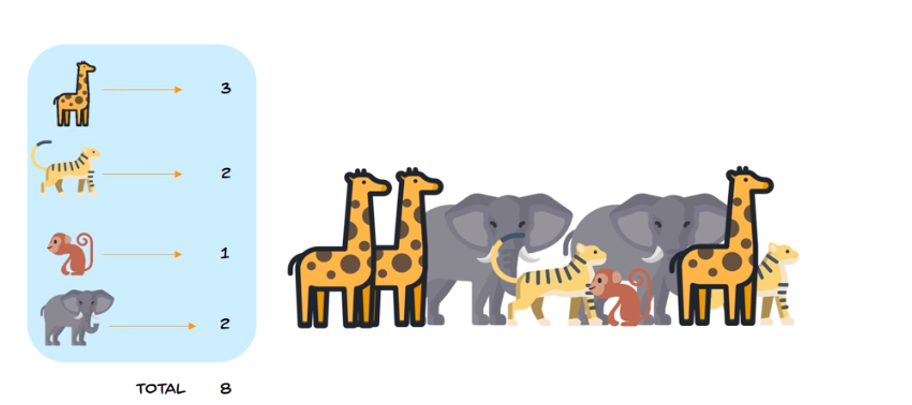Decision Tree Algorithm with all necessary math behind it , Entropy Calculation , Information Gain calculation for a given dataset

## Decision Trees

Decision Tree is a tree shaped algorithm used to determine a course of action. Each branch of the tree represents a possible decision, occurrence or reaction.

### Information Theory:

Information Theoryis the fundamentals of decision trees. In order for us to understand Decision Tree algorithm, we need to understand Information Theory.

The basic idea of information theory is that the “informational value” of a data-set depends on the degree to which the content of the message is surprising or messy. If an event is very probable, it is no surprise (and generally uninteresting) when that event happens as expected; hence transmission of such a message carries very little new information. However, if an event is unlikely to occur, it is much more informative to learn that the event happened or will happen.

For example, there are at-least 3000 varieties of fishes available in both coast of India alone, if we are building a “shark” classifier to identify whether, it is a Shark or not. It is important for us to introduce non-shark images to the datasets as negative samples in-order for the classifier to distinguish between a shark and a non-shark fish. The *entropy *is high only when the mixture of positive samples (shark) and negative samples (non-shark) are equal, implying the data-set has interesting features to learn.

If all images in our data-set are positive(shark), then we don’t learn anything new — no matter how many images of shark it contains.

It is observed that the entropy is maximum when the probabilities are equal.

### Decision Tree:

The basic idea behind a Decision Tree is to break classification down into a set of choices about each entry (i.e. column) in our feature vector. We start at the root of the tree and then progress down to the leaves where the actual classification is made.

For example, lets assume we are on a hiking trip to lake district and the rain is on and off, we need to decide whether to stay indoor or go on a hike.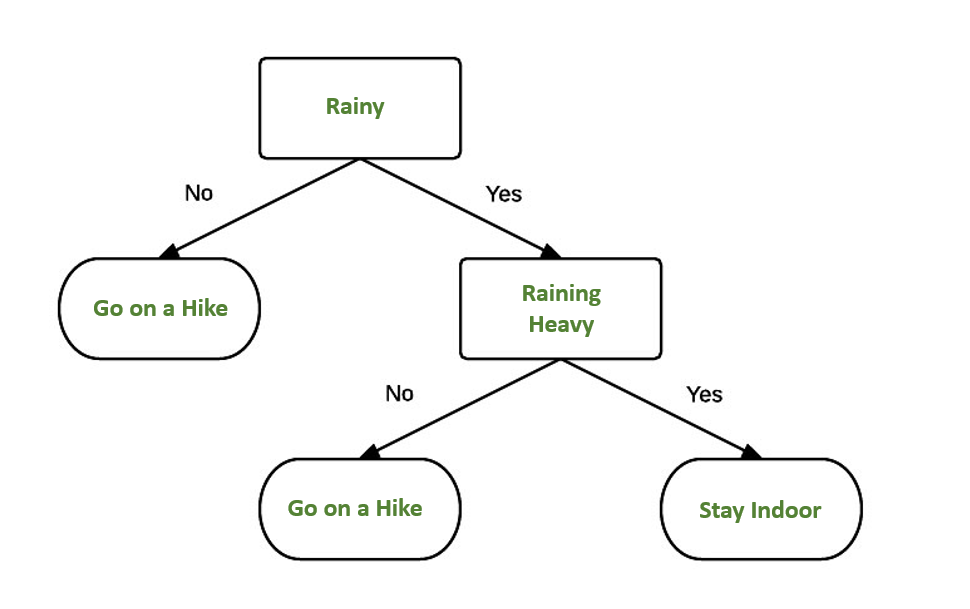FIG 1: AN EXAMPLE OF A DECISION TREE THAT WE CAN USE TO DECIDE IF WE WANT TO GO ON A HIKE OR STAY INDOOR

As you can see from *FIG 1 *above, we have created a decision tree diagram where the decision blocks (rectangles) indicate a choice that we must make. We then have branches which lead us to other decision blocks or a terminating block _(ovals). A _terminating block is a leaf node of the tree, indicating that a final decision has been made.

### Tree Construction:

Decision tree algorithms use information theory in some shape or form to obtain the optimal, most informative splits (i.e. the “decisions”) to construct a series of “if/then” rules in a tree-like manner.

First step in constructing a decision tree is forming the root node of the tree. ie, which feature is split to form the rest of the tree.

In order for us to find the root node, we calculate the information gain from each feature column. The feature column with maximum information gain is selected as the root node and split is made from the selected root node to construct the decision tree.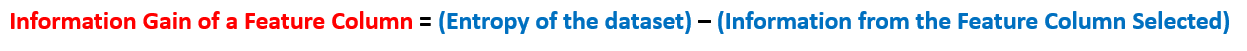Formula to calculate Entropy :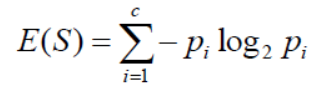Where , p = number of unique values in a feature column / total number of features in a feature column

For example, Lets find the entropy of the below animal dataset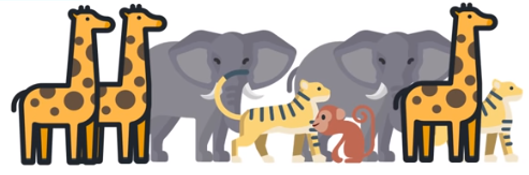FIG 2 : ANIMAL DATASET

The dataset is looking quite messy and the entropy is high in this case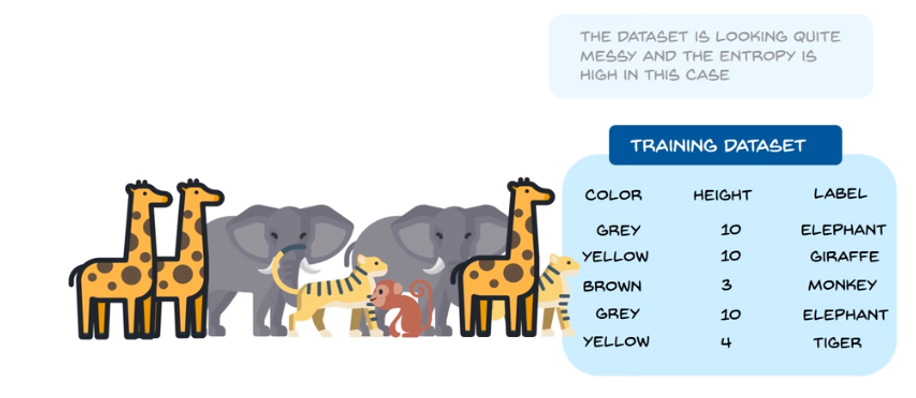Total number of animals= 8

Number of Giraffe in Dataset = 3

Number of Tiger in Dataset = 2

Number of Monkey in Dataset = 1

Number of Elephant in Dataset = 2

Hence the entropy is calculated as below,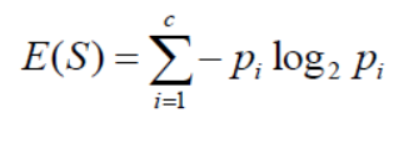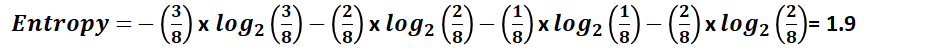``````import math
entropy = -(3/8)*math.log2(3/8)+(2/8)*math.log2(2/8)+(1/8)*math.log2(1/8)+(2/8)*math.log2(2/8)

print(entropy)``````

The entropy here is approximately 1.9. This is considered a high entropy , a high level of disorder ( meaning low level of purity).

## Difference between Machine Learning, Data Science, AI, Deep Learning, and Statistics

In this article, I clarify the various roles of the data scientist, and how data science compares and overlaps with related fields such as machine learning, deep learning, AI, statistics, IoT, operations research, and applied mathematics.

## 15 Machine Learning and Data Science Project Ideas with Datasets

Learning is a new fun in the field of Machine Learning and Data Science. In this article, we’ll be discussing 15 machine learning and data science projects.

## Learn Programming, Software Engineering, Machine Learning, And More

Best Free Resources to Learn Programming, Software Engineering, Machine Learning, And More All you need to learn. Do you know that you can take the courses from MIT, Stanford.

## Most popular Data Science and Machine Learning courses — July 2020

Most popular Data Science and Machine Learning courses — August 2020. This list was last updated in August 2020 — and will be updated regularly so as to keep it relevant

## Statistics for Data Science

Statistics for Data Science and Machine Learning Engineer. I’ll try to teach you just enough to be dangerous, and pique your interest just enough that you’ll go off and learn more.